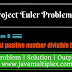## Problem Description :

2520 is the smallest number that can be divided by each of the numbers from 1 to 10 without any remainder. What is the smallest positive number that is evenly divisible by all of the numbers from 1 to 20?

## Concept :

Do you know how we got 2520? Answer is very simple if we will try to get LCM(1,2,3,4,5,6,7,8,9,10) then it is 2520. It means we have to find LCM of all the number from 1 to 20. Now question is how to get LCM of two number in Java? So check below algorithm.

## Algorithm :

We have to perform following steps for finding LCM of two numbers.
1. Get HCM of two numbers because LCM * HCM = product of two numbers. If we will get HCM then we can easily find LCM also.
2. By Euclidean algorithm we can find HCM.
3. We got HCM, so LCM = (product of two numbers) / HCM.

## References :

https://projecteuler.net/problem=5

http://www.math.com/school/subject1/lessons/S1U3L3DP.html

Thank you friends, I hope you have clearly understood the solution of this problem. If you have any doubt, suggestion or query please feel free to comment below. You can also discuss this solution in our forum.

Tags : Project Euler Problem 5, Solution in Java, Smallest Multiple, LCM, HCM, if else statement, while loop, for loop.I am simple guy with lot of ambitions. My main motive is to share whatever knowledge I have related to programming. With me you can easily learn how to solve any programming problem in Java.You can connect with me on social networking sites also.

Project Euler | Problem 5 | Smallest MultipleReviewed by Rohit Agarwal on 12/17/2016 Rating: 5

1.1.2.